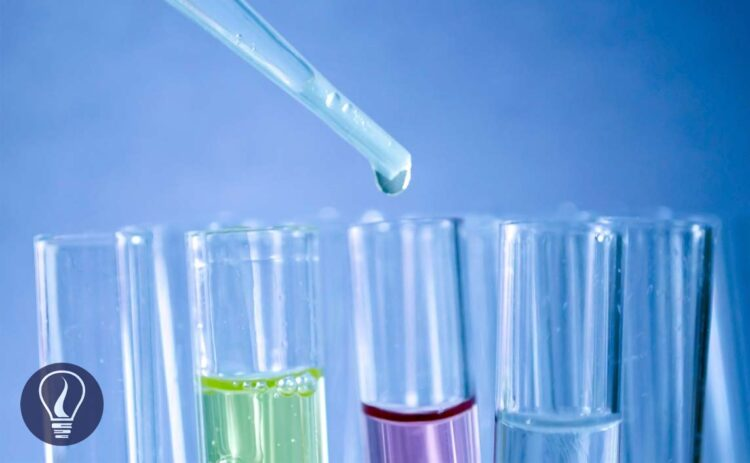More results...

Generic selectors
Exact matches only
Search in title
Search in content
Post Type Selectors

# Ionic Equilibrium - Equilibrium Between Ions and Unionized MoleculesB.Sc (Hons, USJ) (Polymer Science and Technology, Chemistry, Physics)
Categories
Last Updated On : January 20, 2023
Published Date : November 12, 2022

Ionic equilibrium is the equilibrium between ions and unionized molecules. These ions are in a weak electrolyte solution. Strong electrolytes will completely or almost completely dissociate in a solution. So, there are no unionized molecules, and there is no equilibrium. But weak electrolytes do not dissociate completely and show equilibrium.

## Solubility product constant

Solubility product is a kind of equilibrium constant of weak electrolytes in an aqueous medium. The solubility constant only depends on the temperature. Each precipitate is at least slightly soluble in water. Let’s consider a weak electrolyte AxBy which is slightly soluble in water. In an aqueous medium AxBy, the solid will dissociate to ions and show the following equilibrium.

The above system is saturated by Ay+ and Bx- ions at that temperature. The equilibrium constant for the concentration can be written as follows.

The compound AxBy is in a solid state. Its concentration remains constant. Therefore, it is used as a single constant for both Kc and AxBy concentration. This new constant is known as “The solubility product constant (Ksp)”.

### Examples of solubility constant

The solubility of AgCl in water at 25℃ is 1*10-5 mol dm-3. At that temperature, the solubility constant of AgCl can be written as follows,

The solubility of Al(OH)3 in water at a specific temperature is 1.2 * 10-6 mol dm-3. At that temperature, the solubility constant of Al(OH)3 can be written as follows,

The solubility of PbF2 in water at 10℃ is 0.4655 g dm-3. Find the solubility constant of PbF2 at 10℃. (F = 19 amu, Pb = 204 amu)

## Formation of a precipitate

Precipitates are formed after the solution is saturated. If a compound is dissolved in an aqueous medium at its maximum level at a particular temperature, it is called that the solution is saturated by that compound at that temperature. In saturated solutions, the ionic product is equal to the Ksp. For an equilibrium system, the ionic product is given by,

If a saturated solution is added to more compounds, it will no longer be dissolved. The compound will precipitate. When dissolving a compound in an aqueous medium, it is started to form a precipitate, which means the solution has been saturated by that compound.

If the ionic product is lower than the Ksp, there is no precipitate formed. Precipitated will be formed when the ionic product is equal to or higher than the Ksp.

Ksp> ionic product, no precipitate

Ksp <= ionic product, a precipitate is formed

## Common ion effect

When an ionic equilibrium system is added more ions which are also given by the electrolyte in the equilibrium, the solubility of the electrolyte will decrease according to Le Chatelier’s principle. But here, the solubility constant is not going to be changed.

As an example, let’s consider if an equilibrium system of AgCl / Ag+, Cl- is added NaCl. AgCl is partially dissolved in water at room temperature and NaCl is completely dissolved in water. Here the common ion is Cl-.

In the above system, the concentration of Cl- ions is higher. According to Le Chatelier’s principle, the back reaction happens more and more to reduce the Cl- ions in the system. Therefore, the solubility of AgCl is reduced.

### Examples of common ion effect

Find the solubility of AgCl in an aqueous solution of CaCl2 with a concentration of 0.01 mol dm-3. The solubility constant of AgCl at that temperature is 1*10-10 mol2 dm-6

Let’s take the solubility of AgCl2 in CaCl2 solution at that temperature as “x”.

Because there is no temperature change in the system, the solubility constant is not going to be changed.

The solubility of AgCl(s) in CaCl2 solution will be 5×10-9 mol dm-3. If there is no common ion, the solubility can be calculated as follows.

Concentrations of Ag+ and Cl- are equal and let’s take them as “y”. And “y” is the solubility of AgCl at that temperature. so, the Ksp can be written as follows,

The above example shows that the solubility is dramatically reduced due to the common ions.## References and Attributes

### Figures:

The cover image was created using an image by PublicDomainPictures from Pixabay